Disclosure: This post may contain affiliate links, meaning when you click the links and make a purchase, we receive a commission.

# One and Two Ways (Variables) Sensitivity Analysis in Excel!

Sensitivity analysis studies how different sources of uncertainty can affect the final output in a mathematical model.

Recently one of my friends borrowed money from a bank to buy a house. And I was asked to determine how monthly payment will vary as the total amount borrowed or term length change.

At that time, what-if analysis in Excel came to my mind as it allows me to quickly try out different values for formulas.

Here are the details about the mortgage. My friend planned to borrow \$200,000 and the interest rate is 3.40%. The mortgage term is 30 years which means 360 months.

To start off, I only applied one-way data table to see how the changes in mortgage amount will affect the mortgage payment. The Excel file applied just looks like below.

I have the all the necessary inputs – mortgage amount, interest rate and months – in section 1 and data table in section 2.

Initially, I filled “=PMT (C3/12, C4, C2)” into cell C7, and you can see that my friend needs to pay \$886.96 every month.  Then a range of mortgage amount was put down the column B from cell B8 (shown below).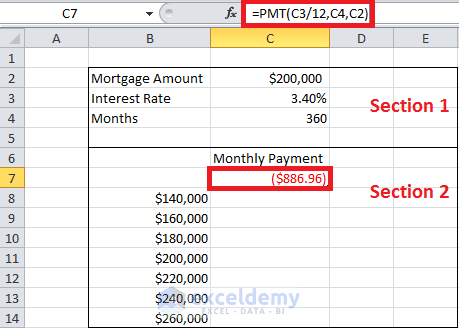Figure 1

## Using one-way (variable) data table () for sensitivity analysis

Now it’s time to create the table.

I selected the table ranges (cells B7: C14 in this case) which begins one row above the first input value (row 7) and the last row is the row containing the last input value (row 14).

The first column in the range is the column containing inputs (column B) and the last column is the column including outputs (column C).  Then clicked the Data tab on the ribbon, chose What-If Analysis and then clicked on Data Table (see below for details).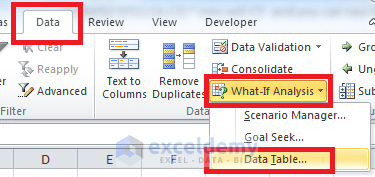Figure 2

A Data Table dialog box (shown below) prompted after clicking on Data Table. Since our table is column-oriented, I just put the column input cell reference – \$C\$2 – and leave the row input cell blank.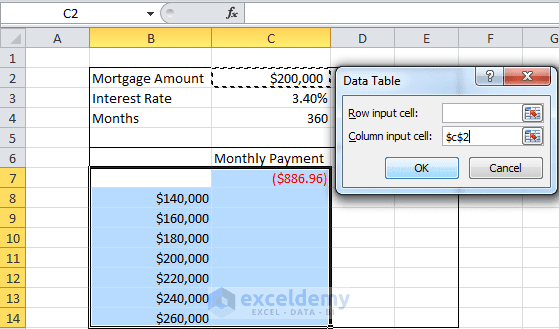Figure 3

By clicking OK, I got below results. When the mortgage amount decreases from \$200,000 to \$140,000, the monthly payment will decrease from \$886.96 to \$620.87. You can also see that the formula for cell C8 is {=TABLE (, C2)}.  This formula is done over the data table range and it will appear in all the cells within the data table range which goes from cell C8 to cell C14.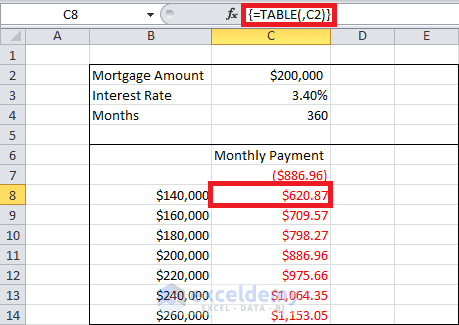Figure 4

I also made a one-way data table to see the impact of changes in term length on the mortgage payment. You can see from below that my friend needs to pay about \$1,419 every month if she plans to pay off in 15 years.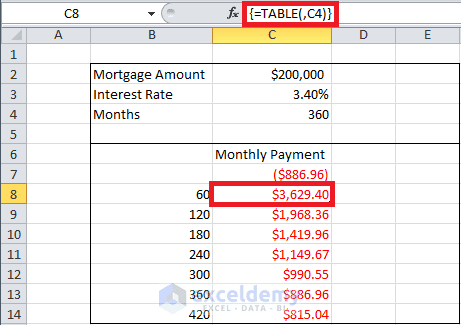Figure 5

## Two-way (variable) data table to analyze the sensitivity

We also would like to know how monthly payment varies as total mortgage varies from \$140,000 to \$260,000 (in \$20,000 increments) and term length varies from 5 years to 35 years (in 5 years increments).

And in this case, we changed two inputs and therefore we created below two-way data table.

We put the mortgage amount values down the first column (Column B) of the table range and term length values in the 7th row.

There is one point that I have to remind you. A two-way data table can have only one output cell, and the formula for the output must be placed in the upper-left corner (B7 in this case) of the table range. Therefore, we placed formula – “=PMT (C3/12, C4, C2)” – in cell B7.

As with one-way table, we selected table range (cells B7: I14) and clicked Data tab. In the Data Tools group, chose What-If Analysis and then select Data Table. Since there are two sources of uncertainty and therefore, I need to fill both rows input cell and column input cell. In our case, cell C2 (mortgage amount) is the column input cell and cell C4 (mortgage term length) is the row input cell.

Thus, the values filled are “\$C\$2” and “\$C\$4” as shown in below screenshot.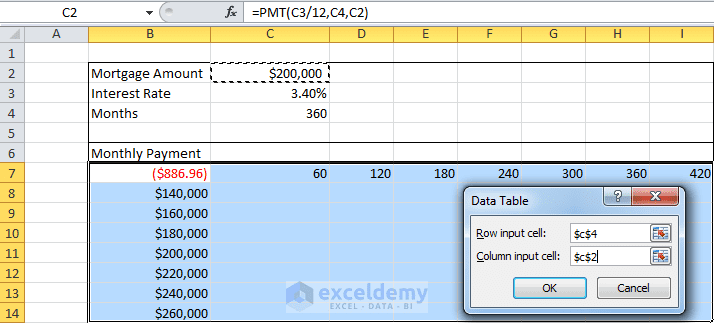Figure 6

After clicking OK, you see the two-way data table shown as following. As an exam­ple, in cell C8, when the mortgage amount is \$140,000 and term length is 5 years or 60 months, the monthly payment equals to \$2,540.58. Did you notice that the formula for cell C8 is {=TABLE (C4, C2)}? This formula is inconsistent with that you filled in Data Table dialog box when compared with above figure, right? You can also compare it with the previous formula ({=TABLE(, C2)}) used in one-way table to figure out the principle and have a deep understanding.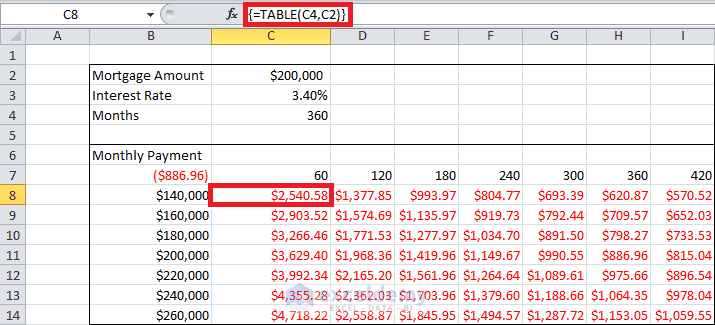Figure 7

## Attention, please! Few notes for you

Finally, I have several notes for you:

• As you change input values in a worksheet, the values calculated by a data table change too. For example, if you increase interest rate from 3.40% to 4.00%, the monthly payment will be \$2578 (number in blue square) given 5 years \$120,000 mortgage. When comparing with above figure, you can see from below screenshot that all values in this two-variable data table have been changed.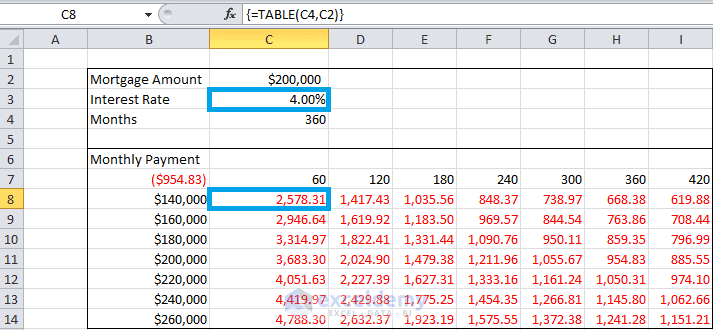• You cannot delete or edit a portion of a data table. If you select on a cell in data table range and edit accidentally, the Excel file will prompt a warning message and you can’t save or change or even close the file anymore. The only way that you can close it is ending the task from task management. It means that your time will be wasted if you did not save the file before making that mistake.
• Though the column oriented one-way table (which is also applied in this post) is used popularly, you can also make your own row oriented one-way table by yourself. Just put inputs in one row and only fill row input cell reference in Data Table dialogue box.
• When making a two-way data table, do not mix up your row input cell and column input cell. As this kind of mistake can result in a big error and nonsensical results.
• The automatic calculation is enabled by default and that’s the reason why any change in the inputs can cause all data in the data table to be recalculated. This is a fantastic feature. However, sometimes, we’d like to disable this feature especially when the data tables are large and automatic recalculation is extremely slow. In this situation, how can you disable automatic calculation? Just click the File tab on the ribbon, choose Options, and then click the Formulas tab, select Automatic Except For Data Tables. Now all your data in data table will be recalculated only when you press the F9 (recalculation) key.